This video was brought to you by GeneralPAC.com, making power systems Intuitive, Open and Free for Everyone, Everywhere. Consider subscribing and supporting through patreon.com/GeneralPAC. This is a mechanism for you to support us financially so we can continue making high quality power system video tutorials. Our corporate sponsor for this topic is AllumiaX.com from Seattle, Washington. Contact them for industrial and commercial power system studies.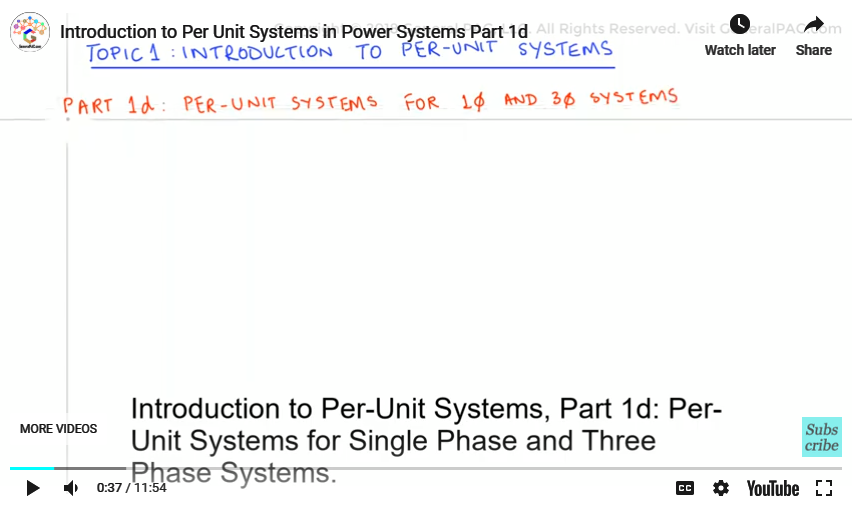In the previous videos, Part 1b and Part 1c, we discussed the formulas for converting values into their per unit equivalents and described base values and then moved forward by solving a simple example. In this video, Part 1c, we will be deriving the formulae that are involved in per unit calculations of single phase and three phase systems, some of those, we have already used in the introductory example in Part 1b and it is essential to have a good understanding on these formulas because we will be using them in calculations in the upcoming videos.

First and foremost, let’s remember that if any two base quantities are given we can find other two base quantities and hence all the four quantities can be known. In electrical power system, the two base quantities that are almost always given are base power, which we denote as S base and base voltage, which we denote as V base. The calculation of other two depends on if we have a single phase system or a three phase system.

Let’s start with the simplest single phase system: We take a source with apparent power, S, and voltage, V, and connected to an impedance Z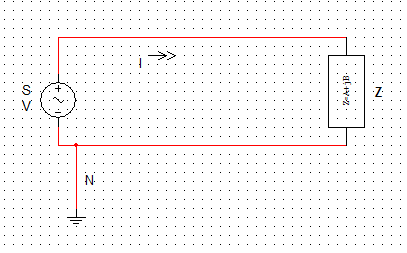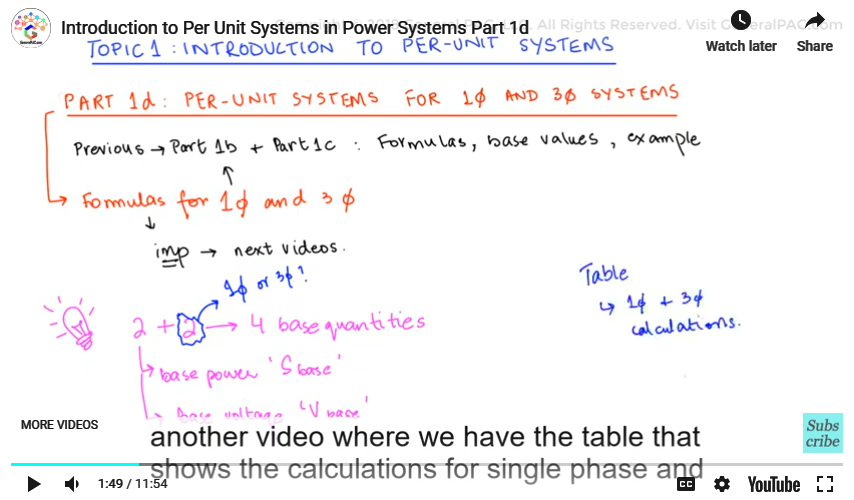The ratings of the source are S and V that are taken as S base and V base. The current flowing is I and the load is Z. this is neutral (point at ground element) since we are dealing with single phase.

We are given S base and V base which are the two known quantities. Let’s calculate I base and Z base which are the unknown but can be easily derived. We know the formula for power that is

Power = Voltage × Current

So,

Base Power = Base Voltage × Base Current but it can be rearranged to the following

Base Current =
Base Power / Base Voltage
(equation #1)
Ibase =
Sbase / Vbase
(Equation #2)

We know the formula for Base impedance is

Base Impedance =
(Base Voltage)2 / Base Power
(Equation #2)
Zbase =
Vbase2 / S base
(Equation #3)

We can see that these all formulas were discussed in Part 1b and we know that per unit values can be calculated using given actual values and these base values.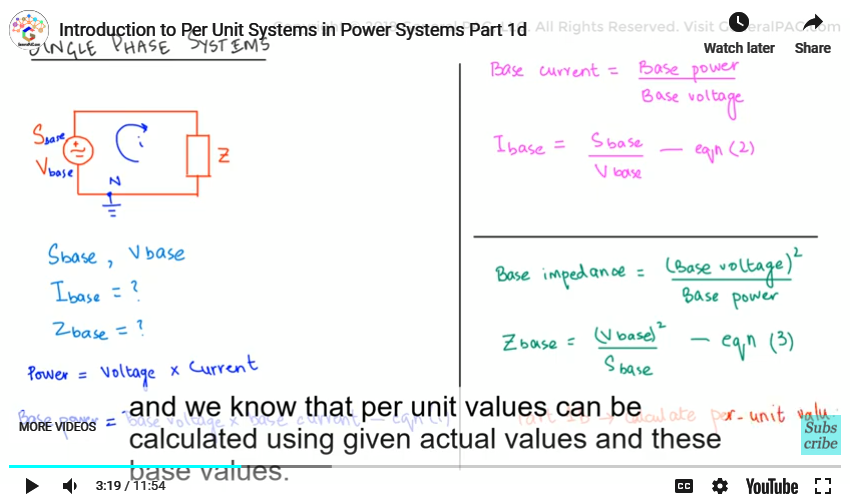Moving on towards three phase systems.

Let’s draw a Y connected system.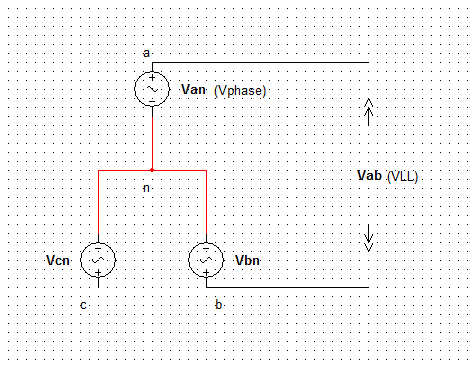‘n’ marked is neutral so,

Van = Vphase or V

And the voltage between a and b is Line to line voltage:

Vab = Vll

Let’s recall some of the basic formulae for Y connected three phase system

Vll =√3 ×V (Equation #4)

Il = I(Equation #5)

S3∅ = 3 × S =3 × V × I(Equation #6)

We will now investigate three key points associated with per unit calculations in three phase systems which will help us understand how we derive the base quantities intuitively.

Keypoint Number 1) Line to line voltage in per unit is always equal to phase voltage in per unit. To prove this let’s look at an example.

It is given that:

Vll(actual) = 100 kV

Vll(base) = 132 kV

So, we will calculate Vll(p.u).

Vll(p.u) =
100 / 132
=0.76 p.u

These were line to line voltages.

Calculating V∅(p.u) requires the actual and base values of voltage of one phase. Let’s convert the line voltage into phase voltage:

V∅(actual) =
100 KV / √3
V∅(base) =
132 KV / √3

So calculating V∅(p.u) by:

V∅(p.u) =
100 ⁄ √3 kV / 132 ⁄ √3 kV
= 100/132=0.76 p.u

Hence it is proved that Line to line voltage in per unit is equal to phase voltage in per unit.

Vll(p.u) = V∅(p.u) (proven)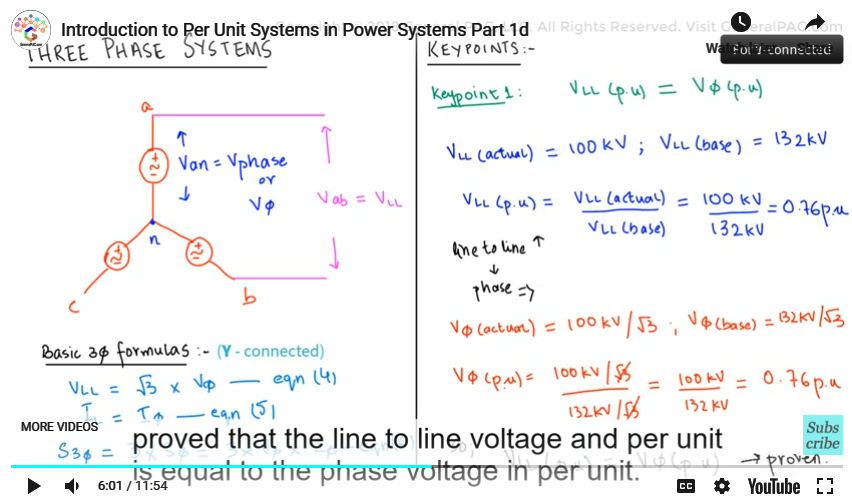Keypoint Number 2) The two base values given are almost always Sbase and Vbase. In three phase systems the Sbase is the total three phase power of the system and Vbase is the line to line voltage. These two quantities are then used to calculate Ibase and Zbase.

Keypoint Number 3) All the base impedances are taken as per phase impedances for three phase systems.

We’ll be looking deeper into Keypoint 2 and Keypoint 3 in the following derivation.

As keypoint 2 states, we are given with Sbase and Ibase in a three phase system. We will now calculate Ibase and Zbase.

Taking Equation #6 that we wrote earlier which is

S3∅ = 3 × S = 3 × V × I

Since S3∅ =S basein a three phase system so:

Sbase =3× V × I

Since we want to calculate base current. the other two quantities should be base power and base voltage. We have Sbase in the above

equation. To incorporate base voltage, we will be Substituting Equation #4 in the above equation which makes:

Sbase = 3 ×
V ll / √3
× I

We know that Vll =V base in a three phase system so:

Sbase = 3 ×
Vbase / √3
× I

Which then simplifies to the following

Sbase = √3 ×
Vbase × I

Then we solve for the phase current which we know as:

I =
Sbase / √3 × Vbase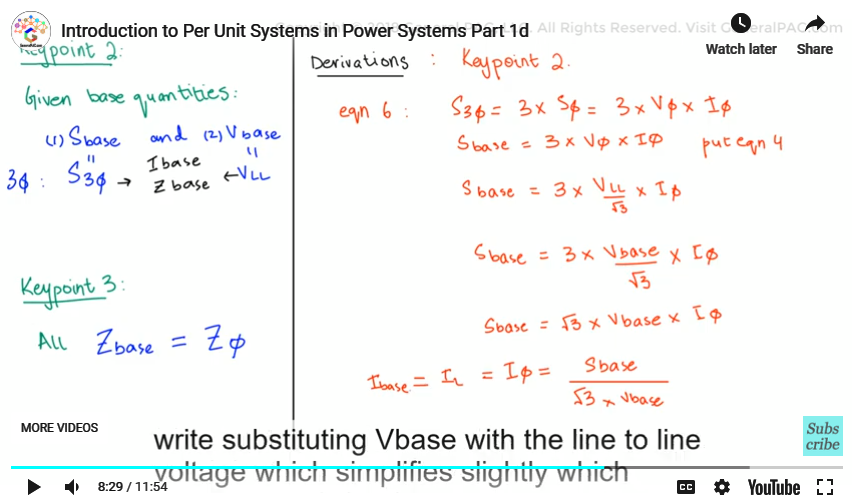We know from eq 5 that I_ll= I_∅ which was taken as Ibase

I= Il = Ibase =
Sbase / √3 × Vbase

Or we can also write, substituting Vbase with Vll

Ibase =
S3∅ / √3 × Vll
(Equation #7)

Here we have the formula for Ibase.

Now let’s calculate Zbase. As keypoint 3 states that all the base impedances are in per phase so

Z =
V / I
(Equation #8)

Substituting eq 4 into eq 8 gives:

Z =
Vll⁄√3 / I

We can also write this equation as:

Zbase =
Vll / √3 / Ibase

Now let’s substitute eq 7 into the above eq:

Zbase =
Vll / √3 / S3∅ ⁄ √3 × Vll

Rearranging this equation:

Zbase =
Vll / √3 × √3 × Vll / S3∅

Root 3 root 3 cancel so it gives us:

Zbase =
Vll2 / S 3∅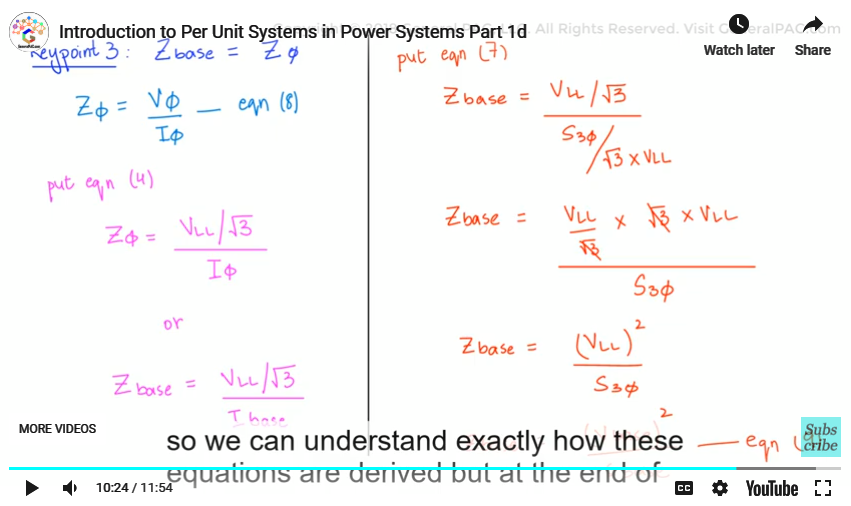or we can also write this as:

Zbase =
Vbase2 / S base
----eq 9

Now we went through his long and complicated derivation so we can understand exactly how these equations are derived… but at the end of the day, we are only concerned about the simplified equations which we will summarize below.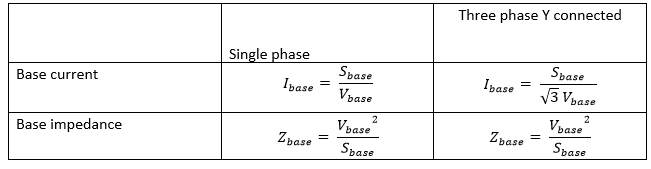These were the formulas that we calculated for single phase and three phase systems These two formulas are same for both single phase and three phase systems provided that V base and S base are VLL and S, three phase respectively for three phase systems.

Zbase =
Vbase2 / S base

In the next part of this series, we’ll be discussing the advantages of per unit systems.

We hope, you have a continued interest in this topic and series as a student or professional. We also hope you find this content useful and enlightening. Please consider subscribing to GeneralPac.com or becoming a patron on patreon.com.

GeneralPAC.com. Making Power Systems Intuitive and free to everyone, anywhere, everywhere.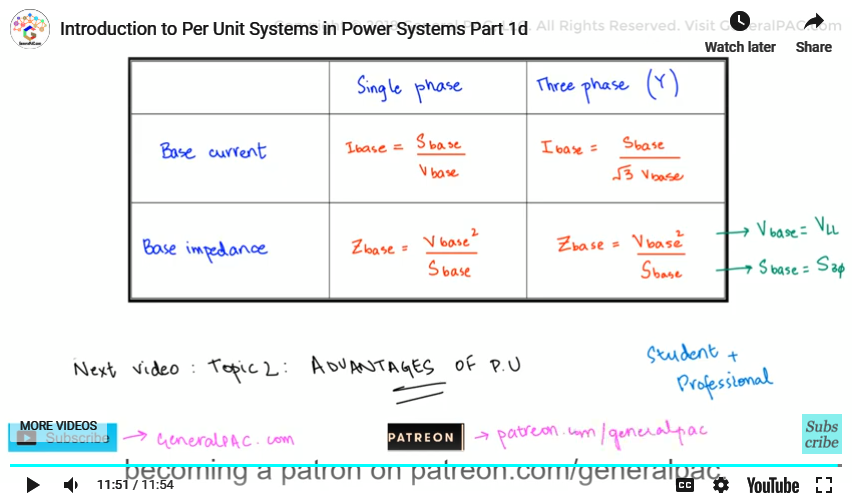#### Greetings from the GeneralPAC Team!

We make high-quality Power Systems Video Tutorials on complex topics that are free and open to everyone!  Thank you so much for supporting us through Patreon so we can continue doing good and valuable work.

What is Patreon and why do we use it?

Patreon is a fantastic portal that allows our fans and community to make monthly contribution (like Netflix subscription) so we can continue creating high-quality power systems video tutorials. In return, you get access to incredible perks like voting on future topics, getting your questions answered, access to VIP Q/A webinars with the creators of GeneralPAC, and much more! We THANK YOU for supporting us

Why do we need your support?

An incredible amount of time and effort is needed to develop high-quality video tutorials. Each video (Part 1 for example) takes approximately 10 hours to complete which includes learning the concept ourselves, brainstorming creative ways to teach and explain the concepts, writing the script, audio recording, video recording, and editing. It's no wonder why Hundreds-of-Thousands of people have watched, liked, subscribed, and left positive comments on Youtube channel. Your support truly makes all the difference.